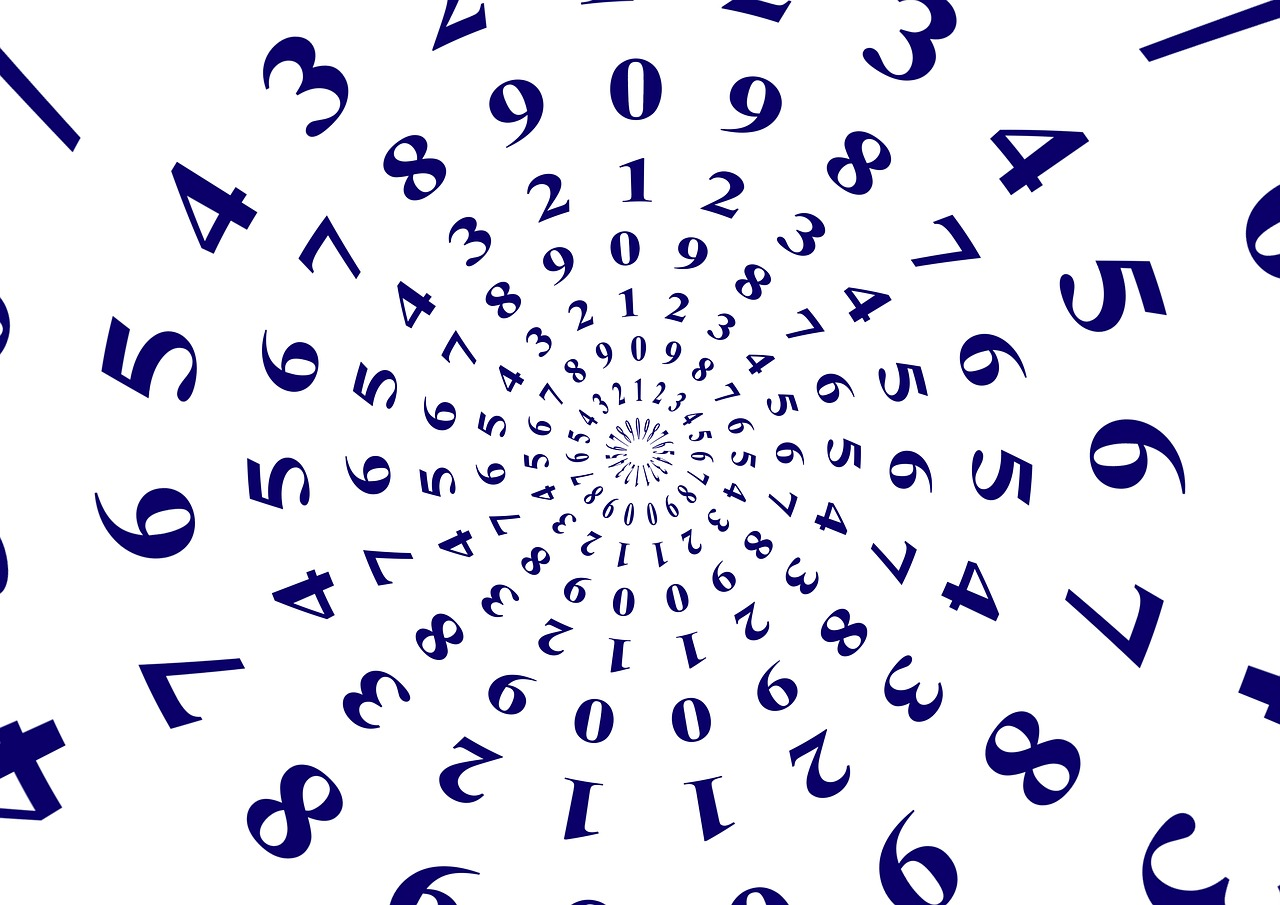# Result of the Day 3 [Problem A] Challenge of my July 2021 Math mini-contest for D.Buzz

in STEMGeeks4 months ago

## Day 3 Problem A

Math mini-contest for D.Buzz for July 2021

A random integer between -200 and +200 is multiplied by a rational number between -2 and +2. The ranges are inclusive. What is the range of the result rounded to the nearest whole number?

The problem was originally posted at https://stemgeeks.net/@savvyplayer/julymathday3a.

## 400 ≥ n ≥ -400

Any of the answers above shall be considered correct.

## Solution

The range in the original problem is -200 ≤ n ≤ 200, where n is the random integer. Multiplying the upper and lower limits of the linear inequality with a negative number will reverse the inequality's upper and lower limits, but since the upper and lower limits are the same number, the sign of the multiplier does not affect the result. If the rational number that is used to multiply the range is -2, then 400 ≥ n ≥ -400. If the rational number used to multiply is 2, then -400 ≤ n ≤ 400.Number circle image from Pixabay

## Winner: none

1 HIVE has been transferred to the prize pool, which will be awarded to the participant with the highest number of correctly-answered problems after all the challenge problems in this Math mini-contest for July 2021 have been concluded.

• @lolxsbudoy's answer of "Between -400 and +400" did not have the "inclusive" or "including the numbers themselves" words, so I could not consider his answer correct. Anyway, thanks to @lolxsbudoy for participating.

Mentions: @jfang003, @holovision, @eturnerx (@eturnerx-dbuzz), @ahmadmanga (@ahmadmangazap), @appukuttan66, @paultactico2, @dkmathstats, and @minus-pi
Special mentions: @dbuzz, @chrisrice, @jancharlest, and @mehmetfix

Posted with STEMGeeks

Sort:

Oh, got the correct numbers but I forgot the word inclusive hahha, fair enough. Thanks savvy.

Thanks for participating and accepting my reason why I didn't accept your answer.

!PIZZA !LUV